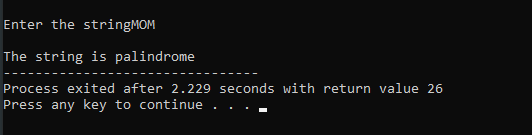# Check if a string palindrome or not using a pointer.

In this program we are going to solve the problem of string palindrome or not using pointer.

## What is a palindrome?

The answer of this question is very important to solve this problem.

Suppose any number like, 121 we reverse that number what will get? The number 121 will get but what our question is, What is a Palindrome number? Yes, the input number after finding the reverse of the number both are same, then that concept is called palindrome number.

Take another example 125 when it reverse we will get 521 which shows this number is not a palindrome number because the input number and after reverse the number is going to be fully changed and returns a new number i.e. 521.

Now, all of you are cleared about palindrome number.

But here the problem is to find the palindrome string through pointer concept in c programming.

## What is a palindrome String?

Like palindrome number the palindrome string plays same role means to reverse the string that will same string at the time of input string.

Likewise, suppose one string “MADAM” when going to be reverse it will return “MADAM” which both are same.

But here is solving this problem we are gone through a pointer concept in c programming.

## What is a pointer variable?

So here is the question is what is a pointer variable? means “Pointer is a variable that stores the address of another variable“.

``````Example:
int a=2,*p;
p=&a;//Here p holds the address of a.``````

Like this way pointer holds the address of string variable.

``````Example:
char str[]="QWERTY",*p;
p = str;//Here p holds the base address of str.``````

Now, we cover all the concepts which are arises while solving this problem.

First, we cover the algorithm of this problem, how to evaluate or check whether a string is a palindrome number or not?

Step-1: Declare the variables like *wrd and *ptr.

Step-2: Register all pointer variables in a memory.

Step-3: Then input the pointer variable(*wrd) with gets() function.

Step-4: Start the while loop assigning characters from one pointer variable(*wrd) to another (*ptr) by help of assignment operator(=).

Step-5: End the *ptr variable with null character(‘\0’).

Step-6: Compare between two pointer variables (*wrd) and (*ptr) if it will returns to 0 then both strings are equal.

And it proves the palindrome of a string.

Now the implementation part of the above algorithm:

```#include<stdio.h>
#include<string.h>
int main()
{
char *wrd,*ptr;
int i,j;
wrd=(char*)malloc(50);
ptr=(char*)malloc(50);
printf("\nEnter the string: ");
gets(wrd);
i=strlen(wrd)-1;
j=0;
while(i>=0)
{
*(ptr+j)=*(wrd+i);
j++;
i--;
}
*(ptr+j)='\0';
if (strcmp(wrd,ptr)==0)
printf("\nThe string is palindrome. ",wrd);
else
printf("\nThe string is not palindrome. ",wrd);
}```

Output:

``````Enter the string: MOM
The string is palindrome.``````Now we briefly discuss,

Here in the above program the string is stored in the pointer variable “wrd”, we find the length and store the address of the string in reverse order in the pointer variable ptr.

The values get stored and the operation keeps on going until the value of i decrements to less than 0.

This is when the loop gets terminated and a NULL ‘\0’ is added at the end of the string in the ptr variable.

Then we compare both the string using strcmp library function found in string.h if it results in 0 then both the strings are the same and hence palindrome, whereas in the else part that is in the false case it represents that the string is not a palindrome.##### Soham Malakar

Hello my name is Soham Malakar am currently pursuing BCA

Coding is one of my favorite hobbies.
I love to code and an enthusiast to learn different types of programming languages.
I love to get intoxicated in the world of technology and video games.
A quick learner possessing better results.

Articles: 40